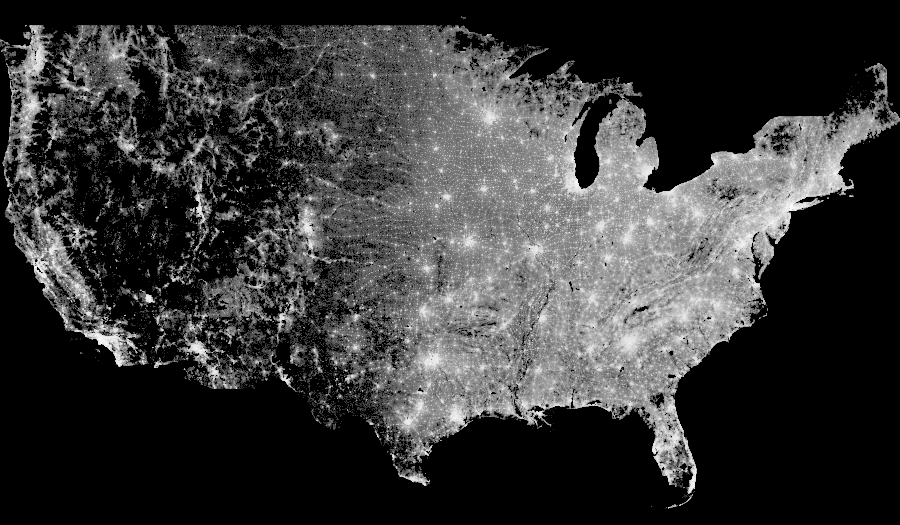Datashader is part of the PyViz initiative for making Python-based visualization tools work well together.

• Datashader is supported and mantained by Anaconda

PyViz

http://pyviz.org/

## US Census¶

```import datashader as ds
import numpy as np
```
```df = dd.io.parquet.read_parquet('/Users/datalab/bigdata/census.snappy.parq')
df = df.persist()
```
```df.head()
```
easting northing race
0 -13700737.0 6275190.0 w
1 -13700711.0 6275195.0 w
2 -13702081.0 6274898.5 w
3 -13701948.0 6274931.0 w
4 -13701793.0 6275088.5 w
```USA           = ((-124.72,  -66.95), (23.55, 50.06))
LakeMichigan  = (( -91.68,  -83.97), (40.75, 44.08))
Chicago       = (( -88.29,  -87.30), (41.57, 42.00))
Chinatown     = (( -87.67,  -87.63), (41.84, 41.86))
NewYorkCity   = (( -74.39,  -73.44), (40.51, 40.91))
LosAngeles    = ((-118.53, -117.81), (33.63, 33.96))
Houston       = (( -96.05,  -94.68), (29.45, 30.11))
Austin        = (( -97.91,  -97.52), (30.17, 30.37))
NewOrleans    = (( -90.37,  -89.89), (29.82, 30.05))
Atlanta       = (( -84.88,  -84.04), (33.45, 33.84))

from datashader.utils import lnglat_to_meters as webm
x_range,y_range = [list(r) for r in webm(*USA)]

plot_width  = int(900)
plot_height = int(plot_width*7.0/12)

background = "black"
```
```from functools import partial
from IPython.core.display import HTML, display

export = partial(export_image, background = background, export_path="export")
cm = partial(colormap_select, reverse=(background!="black"))

display(HTML("<style>.container { width:100% !important; }</style>"))
```
```cvs = ds.Canvas(plot_width, plot_height, *webm(*USA))
agg = cvs.points(df, 'easting', 'northing')
```
```export(tf.shade(agg, cmap = cm(Greys9, 0.2), how='log'),"census_gray_linear")
``````from colorcet import fire